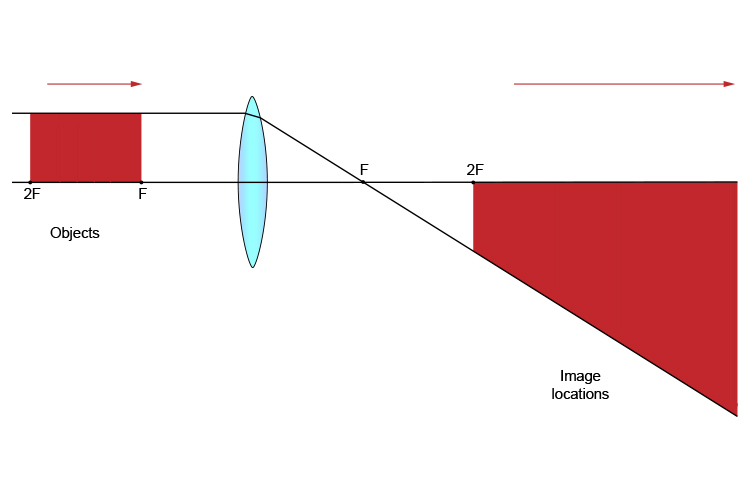# Convex lens – object between 2F and F

This means that the object is between two principal focal lengths and one principal focal length from the convex lens.

As in the previous section, we use the two ray diagram rules.

1. From the object, pass a parallel line through the principal focal point
2. From the object, pass a ray line through the centre of the convex lens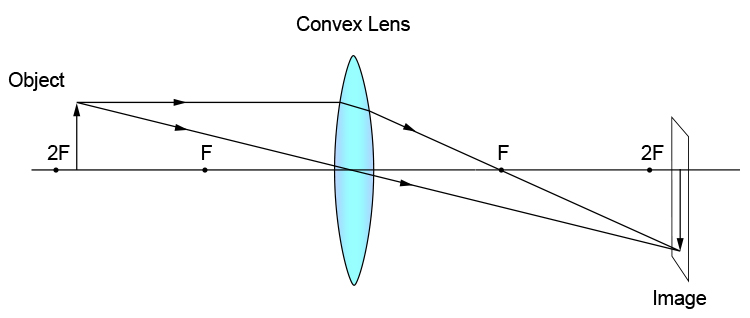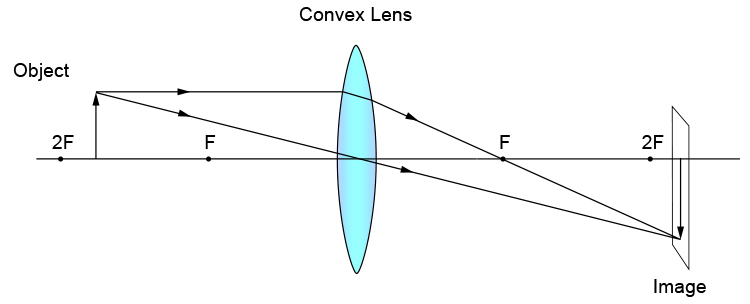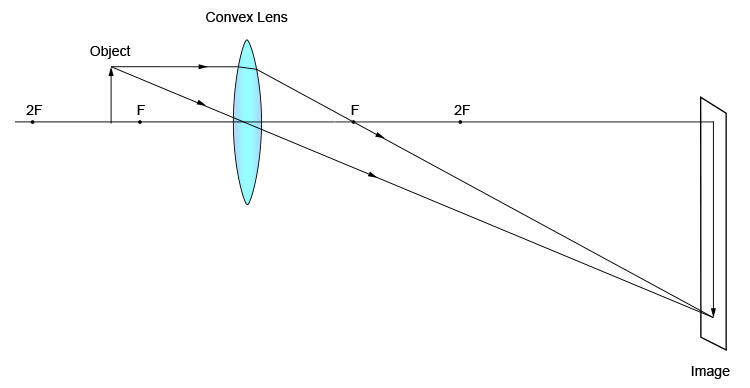From the above diagrams we can observe that all the images are located beyond two principal focal points (2F) on the other side of the convex lens. All the images are larger than the object and can be much larger as they approach one principal focal length (F). All the images are inverted and although you may need a giant screen they can all be focused on that screen.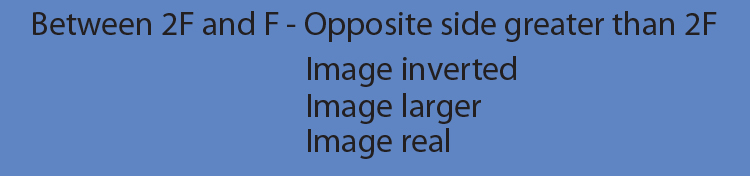In summary this would be: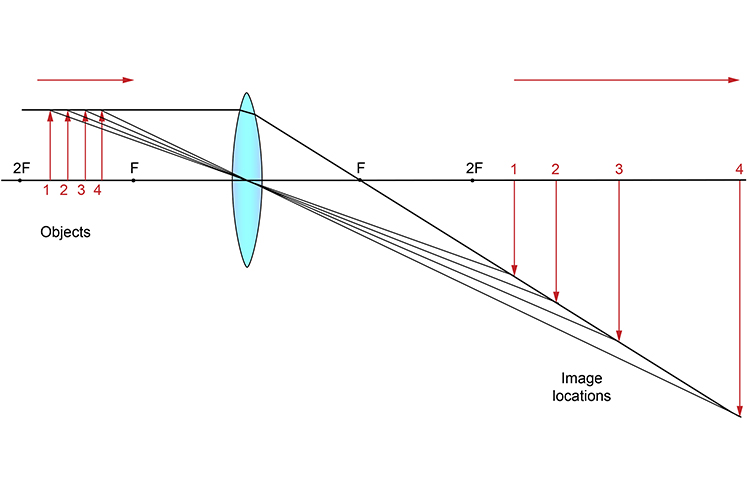Or to make it really simple: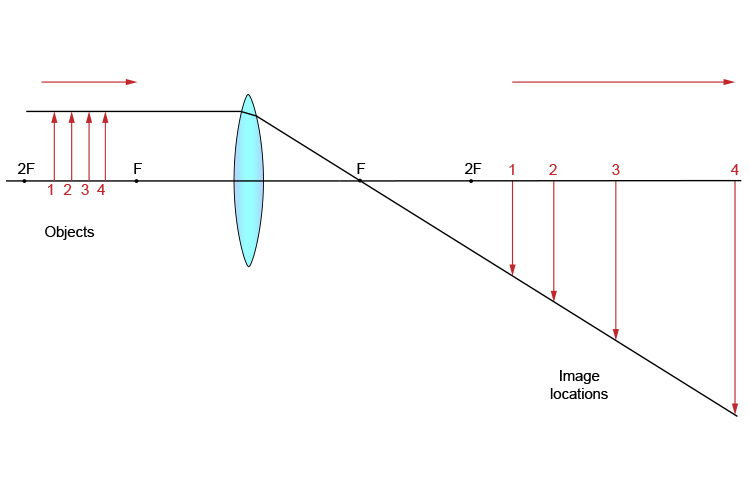Or as a block diagram: